HOME COURSES PREVIEW REVIEW ABOUT CONTACTToll-Free Info & Ordering M-F: 9am-5pm (PST): (877) RAPID-1024/7 Technical SupportQUICK TOURMember Login:Rapid Courses Catalog Mathematics in 24 Hours Chemistry in 24 Hours Biology in 24 Hours Physics in 24 HoursMath Survival Weekly
Get the insider's tips and tricks in how to survive your math course and ace the next test. Subscribe the Web's only math weekly newsletter for students and learn:
- How to Study Math Effectively
- How to Take Math Courses Strategically
- How to Solve Math Problems Systematically
- How to Score High in Math Exams
- How to Master Math Rapidly

Enter your name and email below and get started today!Math Study Lounge These study sheets are for quick review on the subjects. Refer to our rapid courses for comprehensive review.     - Getting Started with Algebra     - Geometry Basics     - How to Solve Math Problems     - Trigonometry Quick Review     - Statistics At-A-Glance     - Calculus PreviewHome » College Algebra

Counting Principles and Basic Probability

 Topic Review on "Title": Fundamental Counting Principle: Suppose two operations are to be performed in order: With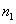possible outcomes for the first, and for each of these there are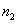possible outcomes for the second. Hence, the total number of possible outcomes is given by the product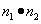. Permutations: A permutation is an ordered arrangement of a set of objects in a row. Combinations: For any natural number, we define. Suppose r objects are selected from a set of n objects without regard to order, each such selection is called a combination, denoted by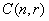or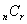. Theorem of Combination: The number of combinations taken r at a time of a set of n objects is given by. Probability: The probability of any event H is the sum of the probabilities of those outcomes of the sample space which belongs to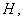denoted byProbability of Successes for H:Theorem I of Overlapping Events: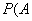orandTheorem II of Disjoint Events: If A and B are mutually exclusive events, thenor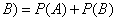. Theorem of Dependent Events: If the probability of an event A depends on the occurrence of an event B, thenand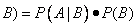wherethe probability that if B has occurs, then A occurs. Theorem of Independent Events: If A and B are independent events thenand.

Rapid Study Kit for "Title":
 Flash Movie Flash Game Flash Card Core Concept Tutorial Problem Solving Drill Review Cheat Sheet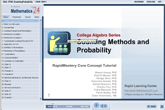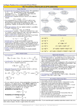"Title" Tutorial Summary : This tutorial shows the principal concepts of probability and counting. Permutations are shown in the examples with the use of tree diagrams and illustrations. The Fundamental Counting Principle is described with the use of an example. Combinations are shown with the use of factorials and tree diagrams. The theorem of combination is shown with the use of examples. The different types of probability distributions are described in this tutorial. The definition and use of probability in application problems are presented in this tutorial with graphical representation and tree diagrams. The probability theorems are introduced with scenarios where they can be utilized. Disjoint and overlapping events are presented along with their probabilities.

 Tutorial Features: Specific Tutorial Features: • Several example problems with step by step illustrations of solutions. • Counting and Basic Probabilities are shown in the example with tree diagrams • Independent and dependent events are given with graphical representations. Series Features: • Concept map showing inter-connections of new concepts in this tutorial and those previously introduced. • Definition slides introduce terms as they are needed. • Visual representation of concepts • Animated examples—worked out step by step • A concise summary is given at the conclusion of the tutorial.

 "Title" Topic List: `Counting Principle and Permutations Fundamental Counting Principle Definition of PermutationsCombinations Factorial Notation Definition and formula of combinationsBinomial Distributions and the Binomial TheoremThe Definition and the use of probability Probability TheoremsProbability of Disjoint and Overlapping Events Theorem I of Overlapping Events Theorem II of Disjoint EventsProbability of Independent and Dependent Events`

See all 24 lessons in College Algebra, including concept tutorials, problem drills and cheat sheets: Teach Yourself College Algebra Visually in 24 Hours

 ©2014 Rapid Learning Center. Privacy Policy | Disclaimer Chemistry Survival Publishing, Biology Survival Publishing and Physics Survival Publishing are the divisions of Rapid Learning Inc.